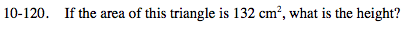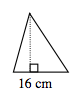### Home > MC2 > Chapter 10 > Lesson 10.2.4 > Problem10-120

10-120.Use the equation for finding the area of a triangle.

$\text{Area} = \frac{1}{2} (\text{base})(\text{height})$

Substitute all the values that are known.

$132 = \frac{1}{2} (16)(\text{height})$

Now simplify and solve for height.